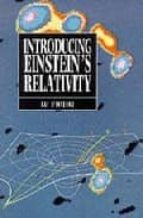# Venta y envío de libros

Venta de libros y envío a todo el mundo

# INTRODUCING EINSTEIN S RELATIVITYISBN: 9780198596530
R.A. D'Inverno  There is little doubt that Einstein's theory of relativity captures the imagination. Not only has it radically altered the way we view the universe, but the theory also has a considerable number of surprises in store. This is especially so in the three main topics of current interest that this book reaches, namely: black holes, gravitational waves, and cosmology. The main aim of this textbook is to provide students with a sound mathematical introduction coupled to an understanding of the physical insights needed to explore the subject. Indeed, the book follows Einstein in that it introduces the theory very much from a physical point of view. After introducing the special theory of relativity, the basic field equations of gravitation are derived and discussed carefully as a prelude to first solving them in simple cases and then exploring the three main areas of application. Einstein's theory of relativity is undoubtedly one of the greatest achievements of the human mind. Yet, in this book, the author makes it possible for students with a wide range of abilities to deal confidently with the subject. Based on the author's fifteen years experience of teaching this subject, this is achieved by breaking down the main arguments into simple logical steps. The book includes numerous illustrative diagrams and exercises (of varying degrees of difficulty), and as a result this book makes an excellent course for any student coming to the subject for the first time.